• 高斯朴素贝叶斯方法进行鸢尾花分类
千次阅读
2020-12-10 01:53:51贝叶斯方法完整代码

import seaborn as sns

X_iris = iris.drop('species', axis=1)

y_iris = iris['species']

print(X_iris)

from sklearn.cross_validation import train_test_split

Xtrain, Xtest, ytrain, ytest = train_test_split(X_iris, y_iris, random_state=1)

from sklearn.naive_bayes import GaussianNB

model = GaussianNB()

model.fit(Xtrain, ytrain)

y_model = model.predict(Xtest)

from sklearn.metrics import accuracy_score

accuracy_score(ytest, y_model)

步骤分析

一-首先获取数据.

这里我们在线导入seaborn库的iris(鸢尾花)数据

import seaborn as sns

这是github上的说明, 可直接下载csv文档

二-将数据格式化

X_iris = iris.drop('species', axis=1)

y_iris = iris['species']

将类别那一列删除生成新的对象赋值给X_iris, y_iris为分类.

pandas的drop方法参考

三-将数据切分为训练数据和测试数据

from sklearn.cross_validation import train_test_split

Xtrain, Xtest, ytrain, ytest = train_test_split(X_iris, y_iris, random_state=1)

cross validation是交叉验证的意思, 参考文章

参数random_state是固定的随机种子, 参考文章

四-调用高斯朴素贝叶斯实现训练

from sklearn.naive_bayes import GaussianNB

model = GaussianNB()

model.fit(Xtrain, ytrain)

y_model = model.predict(Xtest) # 进行预测

调用Gaussian naive Bayes模型, 并进行拟合, 预测

五-对测试结果进行评估

from sklearn.metrics import accuracy_score

accuracy_score(ytest, y_model)

得到准确率为0.9736842105263158

metrics是指标的意思.

说明对特征明显的数据, 即使是非常简单的分类算法也可以高效地进行分析.

更多相关内容
• 朴素贝叶斯算法写的关于鸢尾花分类的程序，有需要的可以自己下载修改使用.
• 在所有的机器学习分类算法中，朴素贝叶斯和其他绝大多数的分类算法都不同。 对于大多数的分类算法，比如决策树,KNN,逻辑回归，支持向量机等，他们都是判别方法，也就是直接学习出特征输出Y和特征X之间的关系，要么是...

在所有的机器学习分类算法中，朴素贝叶斯和其他绝大多数的分类算法都不同。 对于大多数的分类算法，比如决策树,KNN,逻辑回归，支持向量机等，他们都是判别方法，也就是直接学习出特征输出Y和特征X之间的关系，要么是决策函数Y=f(X),要么是条件分布P(Y|X)。 朴素贝叶斯方法是基于贝叶斯定理的一组有监督学习算法，即“简单”地假设每对特征之间相互独立，也就是直接找出特征输出Y和特征X的联合分布P(X,Y),然后用P(Y|X)=P(X,Y)/P(X)得出。 朴素贝叶斯很直观，计算量也不大，在很多领域有广泛的应用 在scikit-learn中，一共有3个朴素贝叶斯的分类算法类。 分别是GaussianNB，MultinomialNB和BernoulliNB。 其中GaussianNB就是先验为高斯分布的朴素贝叶斯， MultinomialNB就是先验为多项式分布的朴素贝叶斯， 而BernoulliNB就是先验为伯努利分布的朴素贝叶斯。 就鸢尾花例子而言，高斯朴素贝叶斯的准确率>多项分布朴素贝叶斯准确率>伯努利朴素贝叶斯准确率，大概和数据的分布情况有关，后续专门再针对性的评估和学习一下 如下：

# 在所有的机器学习分类算法中，朴素贝叶斯和其他绝大多数的分类算法都不同。

# 对于大多数的分类算法，比如决策树,KNN,逻辑回归，支持向量机等，他们都是判别方法，也就是直接学习出特征输出Y和特征X之间的关系，要么是决策函数Y=f(X),要么是条件分布P(Y|X)。

# 朴素贝叶斯方法是基于贝叶斯定理的一组有监督学习算法，即“简单”地假设每对特征之间相互独立，也就是直接找出特征输出Y和特征X的联合分布P(X,Y),然后用P(Y|X)=P(X,Y)/P(X)得出。

# 朴素贝叶斯很直观，计算量也不大，在很多领域有广泛的应用

# 在scikit-learn中，一共有3个朴素贝叶斯的分类算法类。

# 分别是GaussianNB，MultinomialNB和BernoulliNB。

#     其中GaussianNB就是先验为高斯分布的朴素贝叶斯，

#     MultinomialNB就是先验为多项式分布的朴素贝叶斯，

#     而BernoulliNB就是先验为伯努利分布的朴素贝叶斯。

# GaussianNB类参数

#     GaussianNB类的主要参数仅有一个，即先验概率priors，对应Y的各个类别的先验概率P(Y=Ck)。

#         这个值默认不给出，如果不给出此时P(Y=Ck)=mk/m。

#         其中m为训练集样本总数量，mk为输出为第k类别的训练集样本数。

#         如果给出的话就以priors 为准。

#     在使用GaussianNB的fit方法拟合数据后，我们可以进行预测。

#         此时预测有三种方法，包括predict，predict_log_proba和predict_proba。

#         GaussianNB一个重要的功能是有 partial_fit方法，这个方法的一般用在如果训练集数据量非常大，一次不能全部载入内存的时候。

#         这时我们可以把训练集分成若干等分，重复调用partial_fit来一步步的学习训练集

# MultinomialNB类参数

#     MultinomialNB参数比GaussianNB多，但是一共也只有仅仅3个。

#         其中，参数alpha即为上面的常数λ，如果你没有特别的需要，用默认的1即可。

#         如果发现拟合的不好，需要调优时，可以选择稍大于1或者稍小于1的数。

#         布尔参数fit_prior表示是否要考虑先验概率，如果是false,则所有的样本类别输出都有相同的类别先验概率。

#         否则可以自己用第三个参数class_prior输入先验概率，或者不输入第三个参数class_prior让MultinomialNB自己从训练集样本来计算先验概率，此时的先验概率为P(Y=Ck)=mk/m。

#         其中m为训练集样本总数量，mk为输出为第k类别的训练集样本数。

# BernoulliNB类参数

#     BernoulliNB一共有4个参数，其中3个参数的名字和意义和MultinomialNB完全相同。

#         唯一增加的一个参数是binarize。

#         这个参数主要是用来帮BernoulliNB处理二项分布的，可以是数值或者不输入。

#         如果不输入，则BernoulliNB认为每个数据特征都已经是二元的。

#         否则的话，小于binarize的会归为一类，大于binarize的会归为另外一类。

from sklearn.naive_bayes import GaussianNB,MultinomialNB,BernoulliNB

def test_naive_bayes(X_train, X_test, y_train, y_test,X,y):

# 训练数据和测试数据进行标准化

# sc = StandardScaler()

# X_train = sc.fit_transform(X_train)

# X_test = sc.transform(X_test)

scores_train=[]

scores_test=[]

names= ['GaussianNB', 'MultinomialNB', 'BernoulliNB' ]

classifiers = [

GaussianNB(),

MultinomialNB(),

BernoulliNB()

]

h=0.02

X= X[:,:2]

#X = StandardScaler().fit_transform(X)

# X_train = StandardScaler().fit_transform(X_train)

# X_test = StandardScaler().fit_transform(X_test)

X_train, X_test, y_train, y_test = train_test_split(X, y, test_size=.4, random_state=42)

x_min, x_max = X[:, 0].min() - .5, X[:, 0].max() + .5

y_min, y_max = X[:, 1].min() - .5, X[:, 1].max() + .5

xx, yy = np.meshgrid(np.arange(x_min, x_max, h),

np.arange(y_min, y_max, h))

# just plot the dataset first

#cmap_light = ListedColormap(['#AAAAFF', '#AAFFAA', '#FFAAAA'])  # 给不同区域赋以颜色

#cmap_bold = ListedColormap(['#FF0000', '#003300', '#0000FF'])  # 给不同属性的点赋以颜色

cmap_bold = ListedColormap(['#AAAAFF', '#AAFFAA', '#FFAAAA'])  # 给不同区域赋以颜色

cmap_light = ListedColormap(['#FF0000', '#00FF00', '#0000FF'])  # 给不同属性的点赋以颜色

plt.figure(figsize=(10,6))

plt.title("Input data")

plt.scatter(X_train[:, 0], X_train[:, 1], c=y_train, cmap=cmap_light,edgecolors='k')

# Plot the testing points

plt.scatter(X_test[:, 0], X_test[:, 1], c=y_test, cmap=cmap_light, alpha=0.5,edgecolors='k')

plt.xlim(xx.min(), xx.max())

plt.ylim(yy.min(), yy.max())

plt.xticks(())

plt.yticks(())

plt.show()

i=1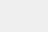figure = plt.figure(figsize=(10, 6))

for name, clf in zip(names, classifiers):

ax = plt.subplot(2, 2, i)

clf.fit(X_train, y_train)

score = clf.score(X_test, y_test)

print('模型名称:{}，得分={}'.format(name,score))

# 模型名称:GaussianNB，得分=0.8333333333333334

# 模型名称:MultinomialNB，得分=0.4666666666666667

# 模型名称:BernoulliNB，得分=0.3

#Z = clf.predict_proba(np.c_[xx.ravel(), yy.ravel()])[:, 1]

Z = clf.predict(np.c_[xx.ravel(), yy.ravel()])

Z = Z.reshape(xx.shape)

ax.contourf(xx, yy, Z, cmap=cmap_bold, alpha=.6)

#plt.pcolormesh(xx, yy, Z, cmap=cmap_bold, alpha=.6)

# Plot the training points

ax.scatter(X_train[:, 0], X_train[:, 1], c=y_train, cmap=cmap_light,edgecolors='k')

# Plot the testing points

ax.scatter(X_test[:, 0], X_test[:, 1], c=y_test, cmap=cmap_light,edgecolors='k', alpha=0.6)

ax.set_xlim(xx.min(), xx.max())

ax.set_ylim(yy.min(), yy.max())

ax.set_xticks(())

ax.set_yticks(())

ax.set_title(name)

ax.text(xx.max() - .3, yy.min() + .3, ('%.2f' % score).lstrip('0'), size=15, horizontalalignment='right')

i=i+1

plt.show()

if __name__=='__main__':

# -----------------------逻辑回归--------------------------------

# test_LogisticRegression(X_train,X_test,y_train,y_test)

# test_LogisticRegression_C(X_train, X_test, y_train, y_test)

# test_LogisticRegression_Cpenaltyssolvers(X_train, X_test, y_train, y_test)

# test_LogisticRegression_Cmulti_classsolvers(X_train, X_test, y_train, y_test)

# test_LogisticRegression_penaltyssolvers(X_train, X_test, y_train, y_test)

# test_LogisticRegression_multi_classssolvers(X_train, X_test, y_train, y_test)

# test_LogisticRegression_best(X_train, X_test, y_train, y_test)

# ---------------------K近邻算法-----------------------

#test_KNeighborsClassifier(X_train, X_test, y_train, y_test, X, y)

# ------------------朴素贝叶斯-----------------------

# 高斯朴素贝叶斯

# 多项分布朴素贝叶斯

# 伯努利朴素贝叶斯

test_naive_bayes(X_train, X_test, y_train, y_test, X, y)

# ---------------------决策树-----------------------

# ---------------------K Means聚类-----------------------

# ------------------高斯混合模型聚类-----------------------

# -------------------SVM支撑向量机-----------------------

展开全文• 朴素贝叶斯处理鸢尾花数据集 朴素贝叶斯公式 上代码 from sklearn import datasets from sklearn.model_selection import GridSearchCV,train_test_split,cross_val_score iris = datasets.load_iris()#下载鸢尾花...

# 朴素贝叶斯处理鸢尾花数据集

朴素贝叶斯公式上代码

from sklearn import datasets
from sklearn.model_selection import GridSearchCV,train_test_split,cross_val_score
data_x = iris["data"]#特征x
# print(data_x)
data_y = iris["target"]#类别y
#将每一列特征划分成几份区间，标准化
num_1=5#每个特征分成五份，我这里是每个特征都分成5个区间，也可以分开。不过代码自己改
def standard_feature(feature_col,num):
max_0 = max(data_x[:,feature_col])
min_0 = min(data_x[:,feature_col])
width_0 = (max_0-min_0)/num
for j in range(len(data_x[:,feature_col])):
for i in range(1, num + 1):
if min_0+(i-1)*width_0<=data_x[j,feature_col]<=min_0+i*width_0:
data_x[j,feature_col] =i
# print(data_x[j,feature])
break
x_col_num = len(data_x)#获取列数及特征数目
def get_pb(one_feature,col,x_train):
'''
one_feature在该x_train数据集的col列的概率
:param one_feature:查找的特征是啥
:param col: 列
:param x_train:
:return: 返回该特征的概率
'''
fea_sum = 0
for i in x_train[:,col]:
if i == one_feature:
fea_sum+=1
col_all = len(x_train)
p_b1 =fea_sum/col_all
# if p_b1 == 0:
#     print("第几列第几个特征个数为0",col,one_feature,len(x_train))#如果当你把x特征分的太细会出现有些特征测试集有但训练集没有。看len(x_train）当这个等于训练集总数第78行会除数为0
return p_b1
for i in range(x_col_num):#data_x将所有特征标准化
standard_feature(i,num_1)
x_train,x_test,y_train,y_test = train_test_split(data_x,data_y,test_size=0.3)#拆分成测试集，训练集
print("训练集样本数量",len(x_train))
print("测试集样本数量",len(x_test))

test_PB_list =[]#
for row_i in range(len(x_test)):
P_B = 1
for col_i in range(x_col_num):
one_pb = get_pb(x_test[row_i,col_i],col_i,x_train)
P_B *= one_pb
#经过for循环得到每个测试集样本的P（B）
test_PB_list.append(P_B)
print("test_PB_list元素个数",len(test_PB_list))
y =3
y_index_list = []#分别存放对应y的全部训练集的全部列索引，该列表元素的列表索引对应y
for y_num in range(y):
one_y_index_list =[]
for y_index in range(len(y_train)):
if y_train[y_index] == y_num:
one_y_index_list.append(y_index)
y_index_list.append(one_y_index_list)
print("训练集中每类拥有索引个数",*[len(a) for a in y_index_list])
y_num_list = []
for y_num in range(y):
one_y_num = 0
for y_index in range(len(y_train)):
if y_train[y_index] == y_num:
one_y_num+=1
y_num_list.append(one_y_num)
print("训练集每类拥有个数",y_num_list)
test_y_predict =[]#测试集预测Y
for test_row in range(len(x_test)):#test_row为测试集每行样本，每行样本都需要计算分类为各个y的概率，哪个概率最大，说明就是哪一类
final_y_P = []
for y_index in range(y):
x_train_yindex = x_train[y_index_list[y_index],:]
P_BinA = 1
for col_i in range(x_col_num):
one_pb = get_pb(x_test[test_row, col_i], col_i, x_train_yindex)
P_BinA *= one_pb
PAinB=(y_num_list[y_index]/len(y_train))*P_BinA/test_PB_list[test_row]
final_y_P.append(PAinB)
belong_class = final_y_P.index(max(final_y_P))
test_y_predict.append(belong_class)
print(test_y_predict)
print(list(y_test))
predict_right_num = 0
for i in range(len(test_y_predict)):
if test_y_predict[i] == y_test[i]:
predict_right_num+=1
probability = predict_right_num/len(test_y_predict)
print("预测正确概率",probability)


接下来打算用其他方法实现分类，以鸢尾花为数据集KNN logistic 决策树

展开全文机器学习 python 算法
• import numpy as np import pandas as pd import matplotlib.pyplot as plt import matplotlib as mpl ...from sklearn.naive_bayes import GaussianNB, MultinomialNB#高斯贝叶斯和多项式朴素贝叶斯 from

贝叶斯原理是怎么来的呢？贝叶斯为了解决一个叫“逆向概率”问题写了一篇文章，尝试解答在没有太多可靠证据的情况下，怎样做出更符合数学逻辑的推测。

什么是“逆向概率”呢？
所谓“逆向概率”是相对“正向概率”而言。正向概率的问题很容易理解，比如我们已经知道袋子里面有 N 个球，不是黑球就是白球，其中 M 个是黑球，那么把手伸进去摸一个球，就能知道摸出黑球的概率是多少。但这种情况往往是上帝视角，即了解了事情的全貌再做判断。
在现实生活中，我们很难知道事情的全貌。贝叶斯则从实际场景出发，提了一个问题：如果我们事先不知道袋子里面黑球和白球的比例，而是通过我们摸出来的球的颜色，能判断出袋子里面黑白球的比例么？
正是这样的一个问题，影响了接下来近 200 年的统计学理论。这是因为，贝叶斯原理与其他统计学推断方法截然不同，它是建立在主观判断的基础上：在我们不了解所有客观事实的情况下，同样可以先估计一个值，然后根据实际结果不断进行修正。
我们用一个题目来体会下：假设有一种病叫做“贝叶死”，它的发病率是万分之一，即 10000 人中会有 1 个人得病。现有一种测试可以检验一个人是否得病的准确率是 99.9%，它的误报率是 0.1%，那么现在的问题是，如果一个人被查出来患有“叶贝死”，实际上患有的可能性有多大？
你可能会想说，既然查出患有“贝叶死”的准确率是 99.9%，那是不是实际上患“贝叶死”的概率也是 99.9% 呢？实际上不是的。你自己想想，在 10000 个人中，还存在 0.1% 的误查的情况，也就是 10 个人没有患病但是被诊断成阳性。当然 10000 个人中，也确实存在一个患有贝叶死的人，他有 99.9% 的概率被检查出来。所以你可以粗算下，患病的这个人实际上是这 11 个人里面的一员，即实际患病比例是 1/11≈9%。
上面这个例子中，实际上涉及到了贝叶斯原理中的几个概念：

先验概率
通过经验来判断事情发生的概率，比如说“贝叶死”的发病率是万分之一，就是先验概率。再比如南方的梅雨季是 6-7 月，就是通过往年的气候总结出来的经验，这个时候下雨的概率就比其他时间高出很多。

后验概率
后验概率就是发生结果之后，推测原因的概率。比如说某人查出来了患有“贝叶死”，那么患病的原因可能是 A、B 或 C。患有“贝叶死”是因为原因 A 的概率就是后验概率。它是属于条件概率的一种。

条件概率
事件 A 在另外一个事件 B 已经发生条件下的发生概率，表示为 P(A|B)，读作“在 B 发生的条件下 A 发生的概率”。比如原因 A 的条件下，患有“贝叶死”的概率，就是条件概率。

似然函数（likelihood function）
你可以把概率模型的训练过程理解为求参数估计的过程。举个例子，如果一个硬币在 10 次抛落中正面均朝上。那么你肯定在想，这个硬币是均匀的可能性是多少？这里硬币均匀就是个参数，似然函数就是用来衡量这个模型的参数。似然在这里就是可能性的意思，它是关于统计参数的函数。
介绍完贝叶斯原理中的这几个概念，我们再来看下贝叶斯原理，实际上贝叶斯原理就是求解后验概率，我们假设：A 表示事件 “测出为阳性”, 用 B1 表示“患有贝叶死”, B2 表示“没有患贝叶死”。根据上面那道题，我们可以得到下面的信息。
患有贝叶死的情况下，测出为阳性的概率为 P(A|B1)=99.9%，没有患贝叶死，但测出为阳性的概率为 P(A|B2)=0.1%。另外患有贝叶死的概率为 P(B1)=0.01%，没有患贝叶死的概率 P(B2)=99.99%。
那么我们检测出来为阳性，而且是贝叶死的概率 P(B1，A）=P(B1)*P(A|B1)=0.01%*99.9%=0.00999%。
这里 P(B1,A) 代表的是联合概率，同样我们可以求得 P(B2,A)=P(B2)*P(A|B2)=99.99%*0.1%=0.09999%。
然后我们想求得是检查为阳性的情况下，患有贝叶死的概率，也即是 P(B1|A)。
所以检查出阳性，且患有贝叶死的概率为：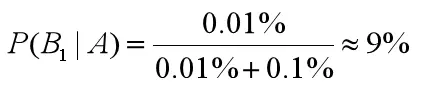检查出是阳性，但没有患有贝叶死的概率为：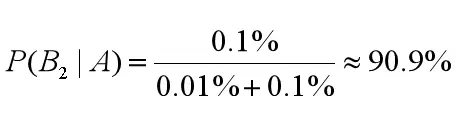这里我们能看出来 0.01%+0.1% 均出现在了 P(B1|A) 和 P(B2|A) 的计算中作为分母。我们把它称之为论据因子，也相当于一个权值因子。
其中 P(B1）、P(B2) 就是先验概率，我们现在知道了观测值，就是被检测出来是阳性，来求患贝叶死的概率，也就是求后验概率。求后验概率就是贝叶斯原理要求的，基于刚才求得的 P(B1|A)，P(B2|A)，我们可以总结出贝叶斯公式为：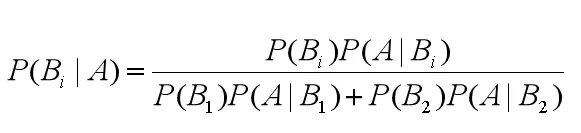由此，我们可以得出通用的贝叶斯公式：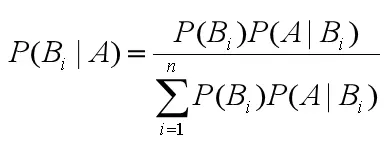下边举个鸢尾花数据分类的例子import numpy as np
import pandas as pd
import matplotlib.pyplot as plt
import matplotlib as mpl
from sklearn.preprocessing import StandardScaler, MinMaxScaler, PolynomialFeatures
from sklearn.naive_bayes import GaussianNB, MultinomialNB#高斯贝叶斯和多项式朴素贝叶斯
from sklearn.pipeline import Pipeline
from sklearn.metrics import accuracy_score
from sklearn.model_selection import train_test_split
from sklearn.neighbors import KNeighborsClassifier

## 设置属性防止中文乱码
mpl.rcParams['font.sans-serif'] = [u'SimHei']
mpl.rcParams['axes.unicode_minus'] = False

# 花萼长度、花萼宽度，花瓣长度，花瓣宽度
iris_feature_E = 'sepal length', 'sepal width', 'petal length', 'petal width'
iris_feature_C = u'花萼长度', u'花萼宽度', u'花瓣长度', u'花瓣宽度'
iris_class = 'Iris-setosa', 'Iris-versicolor', 'Iris-virginica'
features = [2,3]

## 读取数据
path = './datas/iris.data'  # 数据文件路径
x = data[list(range(4))]
x = x[features]
y = pd.Categorical(data).codes ## 直接将数据特征转换为0，1,2
print ("总样本数目：%d；特征属性数目：%d" % x.shape)

## 0. 数据分割，形成模型训练数据和测试数据
x_train1, x_test1, y_train1, y_test1 = train_test_split(x, y, train_size=0.8, random_state=14)
x_train, x_test, y_train, y_test = x_train1, x_test1, y_train1, y_test1
print ("训练数据集样本数目：%d, 测试数据集样本数目：%d" % (x_train.shape, x_test.shape))


训练数据集样本数目：120, 测试数据集样本数目：30

## 高斯贝叶斯模型构建
clf = Pipeline([
('sc', StandardScaler()),#标准化，把它转化成了高斯分布
('poly', PolynomialFeatures(degree=1)),
('clf', GaussianNB())])
## 训练模型
clf.fit(x_train, y_train)


Pipeline(steps=[(‘sc’, StandardScaler(copy=True, with_mean=True, with_std=True)), (‘poly’, PolynomialFeatures(degree=1, include_bias=True, interaction_only=False)), (‘clf’, GaussianNB(priors=None))])

## 计算预测值并计算准确率
y_train_hat = clf.predict(x_train)
print ('训练集准确度: %.2f%%' % (100 * accuracy_score(y_train, y_train_hat)))
y_test_hat = clf.predict(x_test)
print ('测试集准确度：%.2f%%' % (100 * accuracy_score(y_test, y_test_hat)))



训练集准确度: 95.83%
测试集准确度：96.67%

## 产生区域图
N, M = 500, 500     # 横纵各采样多少个值
x1_min1, x2_min1 = x_train.min()
x1_max1, x2_max1 = x_train.max()
x1_min2, x2_min2 = x_test.min()
x1_max2, x2_max2 = x_test.max()
x1_min = np.min((x1_min1, x1_min2))
x1_max = np.max((x1_max1, x1_max2))
x2_min = np.min((x2_min1, x2_min2))
x2_max = np.max((x2_max1, x2_max2))

t1 = np.linspace(x1_min, x1_max, N)
t2 = np.linspace(x2_min, x2_max, N)
x1, x2 = np.meshgrid(t1, t2)  # 生成网格采样点
x_show = np.dstack((x1.flat, x2.flat)) # 测试点

cm_light = mpl.colors.ListedColormap(['#77E0A0', '#FF8080', '#A0A0FF'])
cm_dark = mpl.colors.ListedColormap(['g', 'r', 'b'])
y_show_hat = clf.predict(x_show)                  # 预测值
y_show_hat = y_show_hat.reshape(x1.shape)

## 画图
plt.figure(facecolor='w')
plt.pcolormesh(x1, x2, y_show_hat, cmap=cm_light)     # 预测值的显示
plt.scatter(x_train[features], x_train[features], c=y_train, edgecolors='k', s=50, cmap=cm_dark)
plt.scatter(x_test[features], x_test[features], c=y_test, marker='^', edgecolors='k', s=120, cmap=cm_dark)
plt.xlabel(iris_feature_C[features], fontsize=13)
plt.ylabel(iris_feature_C[features], fontsize=13)
plt.xlim(x1_min, x1_max)
plt.ylim(x2_min, x2_max)
plt.title(u'GaussianNB对鸢尾花数据的分类结果, 正确率:%.3f%%' % (100 * accuracy_score(y_test, y_test_hat)), fontsize=18)
plt.grid(True)
plt.show()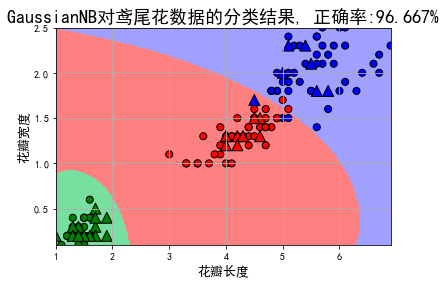链接: 贝叶斯算法例子代码.

展开全文机器学习 python
• 莺尾花数据集–贝叶斯分类 Step1: 库函数导入 import warnings warnings.filterwarnings('ignore') import numpy as np # 加载莺尾花数据集 from sklearn import datasets # 导入高斯朴素贝叶斯分类器 from sklearn....python 机器学习
• 对于这样一个简单的三分类问题，我打算直接采用2.5:7.5的划分并用贝叶斯跟决策树分别训练对比分类效果，详细步骤如下：python 机器学习 决策树
• 第一个应用：鸢尾花分类需要的几个python库scikit-learn：两个 Python 包：NumPy 和 SciPy。NumPy包括多维数组、高级数学函数(比如线性代数运算和傅里叶变换)，以及伪随机数生成器。SciPy具有线性代数高级程序、数学...
• import pandas as pdfrom sklearn.naive_bayes import GaussianNBfrom sklearn.model_selection import train_test_splitfrom sklearn.metrics import accuracy_scorefrom sklearn import datasetsiris=datasets.lo....
• 鸢尾花数据集–贝叶斯分类 Step1：库函数导入 import warnings warnings.filterwarnings('ignore') import numpy as np # 加载莺尾花数据集 from sklearn import datasets # 导入高斯朴素贝叶斯分类器 from sklearn....机器学习 python
• 前面我们已经详细介绍过鸢尾花数据集以及sklearn的使用，下面我们就来调用sklearn中的贝叶斯分类器来实现鸢尾花数据集的分类。 首先，我们需要导入需要用到的包，模型以及数据集 import numpy as np # 导入sklearn...机器学习 分类算法
• 朴素贝叶斯算法原理及鸢尾花分类代码实现 一、概率模型 1、全概率公式(由因推果) 设事件B1,B2,…,Bn构成一个完备事件组,即两两不相容，和为全集且P(Bi)>0,则对任意事件A，有 由因推果的理解：如上图：可以理解...机器学习 python
• (3) 使用朴素贝叶斯处理鸢尾花数据集分类 二、实验内容 （1）导入库，加载鸢尾花数据，输出样本和鸢尾花特征 （2）数据分割，数据分割，形成模型训练数据和测试数据 （3）高斯贝叶斯模型构建 （4）计算预测值并计算...
• 利用决策树，KNN和朴素贝叶斯三种分类器，对鸢尾花数据集进行分类。下面是具体的流程和代码：1、 数据读取：实验数据是直接加载的sklearn内置的鸢尾花数据集，共150条数据，包含4个特征，而且是一个三分类问题。from...
• 常规操作： import numpy as np import ...min, x2_max) plt.title(u'GaussianNB对鸢尾花数据的分类结果, 正确率:%.3f%%' % (100 * accuracy_score(y_test, y_test_hat)), fontsize=18) plt.grid(True) plt.show()
• 基于贝叶斯鸢尾花数据进行分类 1. python3.7 2. numpy >= '1.16.4' 3. sklearn >= '0.23.1' # import base package import warnings warnings.filterwarnings('ignore') import numpy as np from ...
• 数据包含5列，分别是花萼长度、花萼宽度、花瓣长度、花瓣宽度、鸢尾花种类。 鸢尾花属种类包含三种：iris-setosa, iris-versicolour, iris-virginica。 每一类分别是50条，共150条数据；每一类在四个属性的分布情况...
•python
• 鸢尾花卉数据集，是一类多重变量分析的数据集。通过花萼长度，花萼宽度，花瓣长度，花瓣宽度4个属性预测鸢尾花卉属于（Setosa，Versicolour，Virginica）三个种类中的哪一类。是机器学习基础学习的典型案例。
• #导入所需要的包 from sklearn.naive_bayes import GaussianNB import numpy as np import pandas as pd from pandas import Series,DataFrame import matplotlib.pyplot as plt from sklearn.datasets import load_...
• 使用高斯朴素贝叶斯鸢尾花数据进行分类 代码： 1 # 通过朴素贝叶斯鸢尾花数据进行分类 2 3 from sklearn import datasets 4 from sklearn.model_selection import train_test_split 5 from ...
• 主要介绍了python KNN算法实现鸢尾花数据集分类，文中通过示例代码介绍的非常详细，对大家的学习或者工作具有一定的参考学习价值，需要的朋友们下面随着小编来一起学习学习吧
•机器学习 python 人工智能
• 我选用了一个经典数据集来展示如何构建一个决策树模型，这个数据集是——Iris 鸢尾花数据集。里面有我进行数据预处理，分析，优化参数，训练模型以及最终分析决策树的代码。
• 朴素贝叶斯模型是一种基于贝叶斯定理与特征条件独立假设的分类方法， 是经典的机器学习算法之一，也是为数不多的基于概率论的分类算法。 优点：算法逻辑简单,易于实现 缺点：朴素贝叶斯模型前提是假设属性之间...python 机器学习 分类
• sklearn(scikit-learn)是python机器学习常用的第三方模块，是一个开源的机器学习库，它支持监督学习和非监督学习。它还为模型拟合、数据预处理、模型选择和评估以及许多其他实用工具提供了各种工具。...
• 使用SVM支持向量机进行鸢尾花分类_Python实现-附件资源
• knn的python代码 #样本数据150*4二维数据，代表150个样本， #每个样本4个属性分别为花瓣和花萼的长、宽代码
• 朴素贝叶斯 朴素贝叶斯的介绍 朴素贝叶斯算法（Naive Bayes, NB) 是应用最为广泛的分类算法之一。它是基于贝叶斯定义和特征条件独立假设的分类器方法。由于朴素贝叶斯法基于贝叶斯公式计算得到，有着坚实的数学基础...机器学习
• 【python机器学习】逻辑回归算法实现（基于鸢尾花数据集）-附件资源...